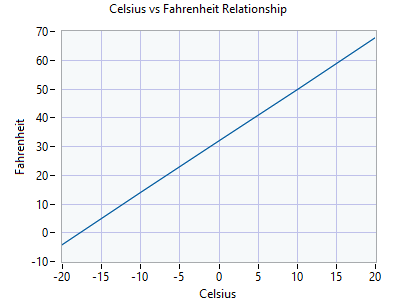top of page
Search

# Deep Learning with LabVIEW. Tutorial #1.3: Linear Regression - Celsius to Fahrenheit Conversion

Updated: Aug 24

## Introduction

Regression Artificial Neural Networks predict continuous numerical values as a function of the inputs. Linear regression used to predict the linear relationship between inputs and outputs. The difference between logistic and linear regressions is that logistic regression predicts probabilities for binary classification, while linear regression assumes a linear relationship between variables, and aims to find the best-fitting line that describes the relationship.

In this blog post, we will consider one of the simplest linear regression problems, i.e. Celsius to Fahrenheit conversion and show how to solve this simple problem with DeepLTK.

If you are not familiar with regression fundamentals and concepts of using DeepLTK, the following articles may be helpful:

This and other DeepLTK based LabVIEW examples can be accessed from our GitHub page.

The project of this tutorial consists of two main VIs:

• 1_Celsius_To_Fahrenheit(Training).vi

• 2_Celsius_To_Fahrenheit(Inference).vi.

## Training: 1_Celsius_To_Fahrenheit(Training).vi.

### Front Panel of the Training VI

Front Panel of the training VI exposes all necessary high level configuration parameters and displays loss function for monitoring the training process.Front Panel of Training VI

### Block Diagram of The Training VIBlock Diagram of Training VI

#### Dataset Generation

In order to train a model we would need a dataset which should contain set of temperature values in Celsius units and their corresponding values Fahrenheit units.

Note: Dataset size used here is arbitrarily chosen to be 5. One can show that if the relationship between input and output is absolutely linear, only two samples (points) in dataset should be enough to fit a model precisely.

Let's analyze the relationship between the input and output.

As we're aware, the Celsius to Fahrenheit conversion formula is F = 5/9*​C + 32. This is a linear relationship of a general form y = k*x + b. So, basically we would need to train a model which should find corresponding k (intercept) and b (bias) values.##### Model SelectionKnowing about the linear relationship between variables, we can solve the problem using just a single neuron. Single neuron model conforms with a linear model presented above, where k intercept is a represented with help of w weight of the neuron, and b is the bias of the same neuron. We will use Fully Connected (FC) and configure its size as 1 (single neuron in a layer). To make sure our chosen model maintains its linearity, we will set the activation function to None.

## Training the Network

Let's run the training VI. After running the VI we can see that the loss value starts to decrease rapidly. After few seconds of training the loss reaches values close to 10e-9 which is enough to assume that the model is perfectly trained, and we can stop the training process.## Inference: 2_Celsius_To_Fahrenheit(Inference)

Now lets test on some samples to evaluate the accuracy of the model.Front Panel of Inference VI

The Front Panel provides two inputs for sourcing .cfg and .bin files (which should be provided from training stage), one numeric control (C) for providing test input values to the network and two numeric indicators F(Prediction) and F(Ground Truth) indicating the trained network's prediction and ground truth values for the specific input.

Supply the necessary .cfg and .bin files, then run the VI. Type specific values in C control for temperatures in Celsius units and observe the network's output at F(Prediction) indicator compared with F(Ground Truth) values.

One can see that a single neuron can perfectly model linear relationship between Celsius and Fahrenheit temperature values.

## Summary

In this blog post, we showcased the usage for for DeepLTK building and training a neural network with a single neuron to perform Celsius to Fahrenheit unit conversions.

In the next blog post we will delve into the understanding of non-linear regression models, where the difference is that non-linear regression deals with more complex, non-linear relationships between variables.

## Things to Do Next

To strengthen the knowledge of readers, we suggest to solve the following similar problems based on the reference example.

Now we recommend to create a model which would do reverse conversion, i.e. convert values from Fahrenheit to Celsius.